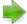#Pillow-Problems: Problem #10

Math Lair Home > Source Material > Pillow-Problems > Problem #10Problem #8 | Problem #12For more information on this collection, see Pillow-Problems by Charles L. Dodgson (Lewis Carroll).

Note: For information on pre-decimal British currency and coins, see pre-decimal British currency.

## Problem:

10. (20, 36)

A triangular billiard-table has 3 pockets, one in each corner, one of which will hold only one ball, while each of the others will hold two. There are 3 balls on the table, each containing a single coin. The table is tilted up, so that the balls run into one corner, it is not known which. The ‘expectation“, as to the contents of the pocket, is 2/6. What are the coins?

[8/90

10. (3, 36)

Either 2 florins and a sixpence; or else a half-crown and 2 shillings.

## Solution:

10.

Call them x, y, z; and let x + y + z = s.

The chance, that the pocket contains 2 balls, is ⅔; and, if it does, the ‘expectation’ is the average value of

(y + z), (z + x), x + y); i.e. it is 2s3.

Also the chance, that it contains only one, is ⅓; and, if it does, the ‘expectation’ is s3.

Hence total ‘expectation’ = 4s9+ s9= 5s9.

5s9 = 30d;   ∴ s = 54d = 4/6.

Hence the coins must be 2 florins and a sixpence; or else a half-crown and 2 shillings.

Q.E.F.